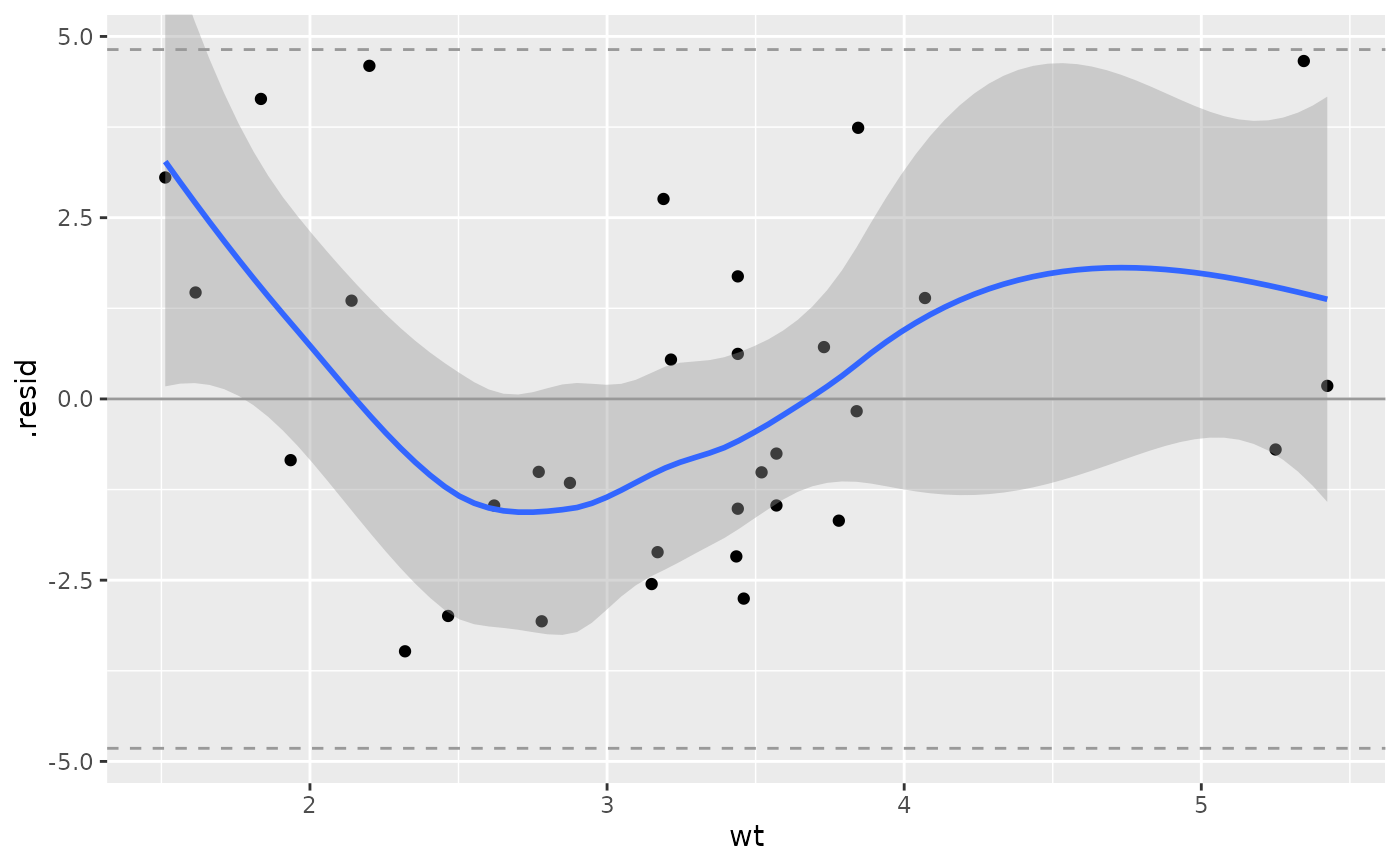If non-null pVal and sigma values are given, confidence interval lines will be added to the plot at the specified pVal percentiles of a N(0, sigma) distribution.

ggally_nostic_resid(
data,
mapping,
...,
linePosition = 0,
lineColor = brew_colors("grey"),
lineSize = 0.5,
lineAlpha = 1,
lineType = 1,
lineConfColor = brew_colors("grey"),
lineConfSize = lineSize,
lineConfAlpha = lineAlpha,
lineConfType = 2,
pVal = c(0.025, 0.975),
sigma = attr(data, "broom_glance")\$sigma,
se = TRUE,
method = "auto",
formula = y ~ x
)

## Arguments

data, mapping, ... parameters supplied to ggally_nostic_line parameters supplied to ggplot2::geom_line() parameters supplied to the confidence interval lines percentiles of a N(0, sigma) distribution to be drawn sigma value for the pVal percentiles boolean to determine if the confidence intervals should be displayed parameters supplied to ggplot2::geom_smooth(). Defaults to "auto" and "y ~ x"

## Value

ggplot2 plot object

stats::residuals
# Small function to display plots only if it's interactive
#> geom_smooth() using method = 'loess'